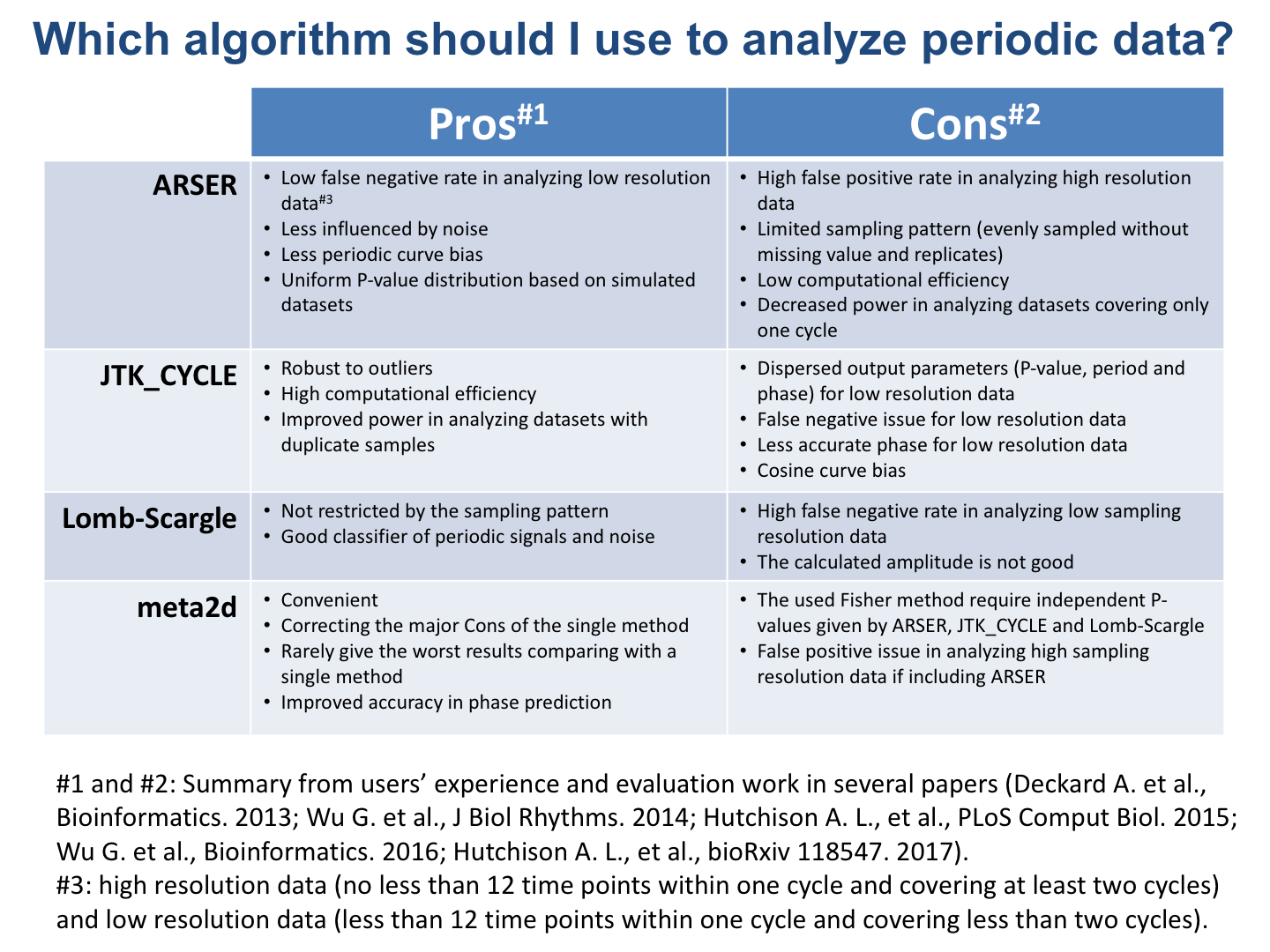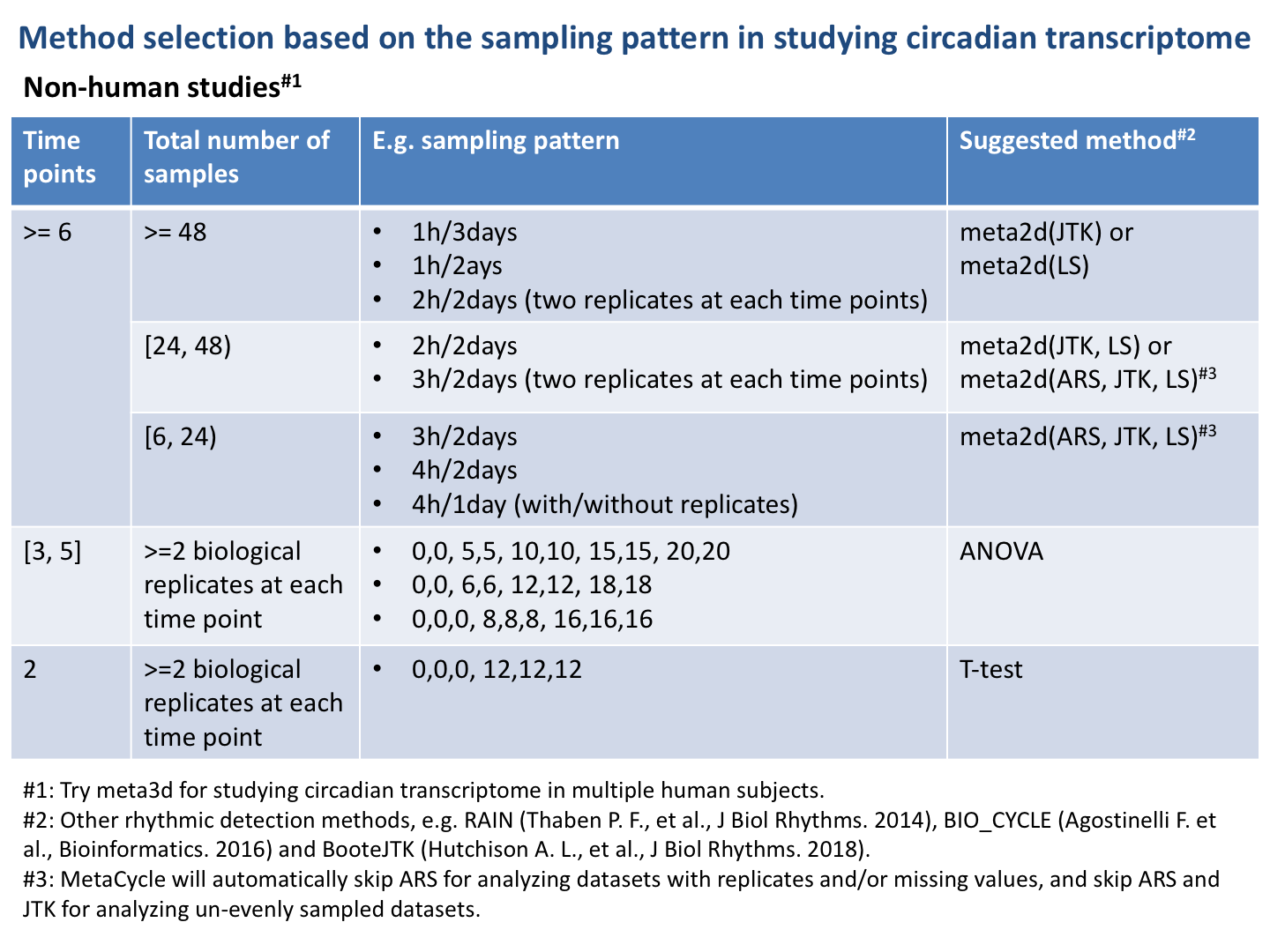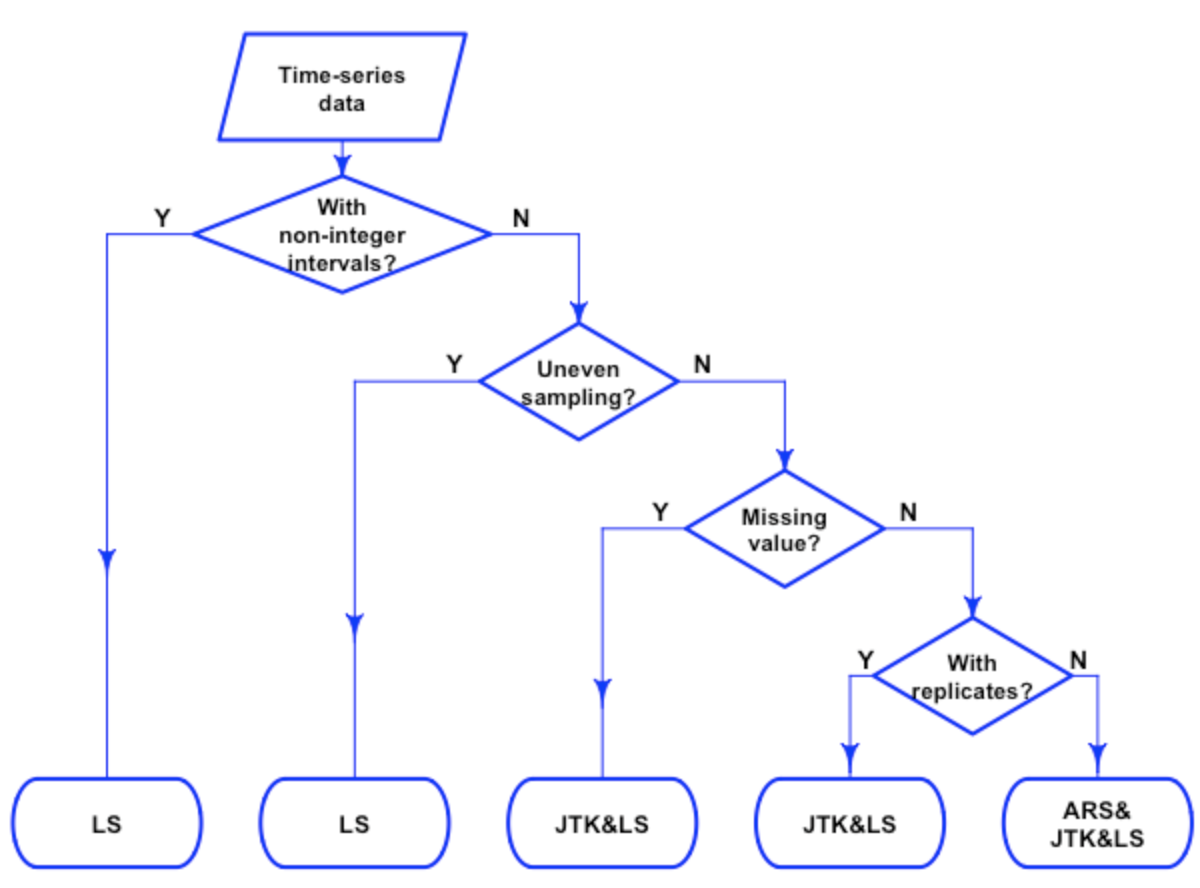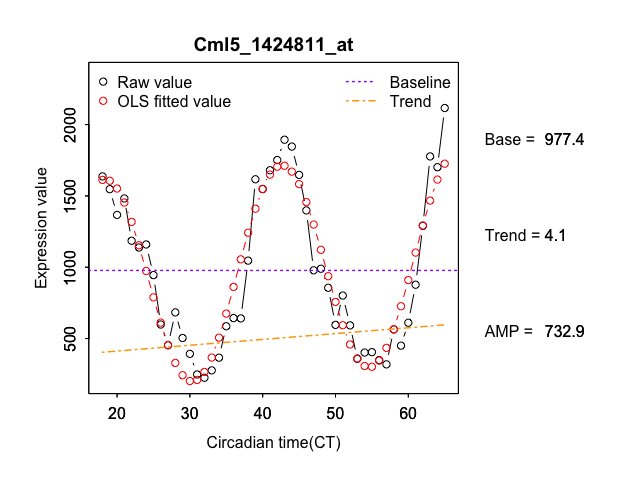# Introduction to MetaCycle

#### 2019-04-18

The MetaCycle package is mainly used for detecting rhythmic signals from large scale time-series data. Depending on features of each time-series data, MetaCycle incorporates ARSER(ARS), JTK_CYCLE(JTK), and Lomb-Scargle(LS) properly for periodic signal detection, and it could also output integrated analysis results if required.

This vignette introduces implementation of method selection and integration steps of MetaCycle, which are not explained in detail in help files. For looking at how to use two main functions–meta2d and meta3d of this package, please see ‘Examples’ part of each function’s help file.

The MetaCycle source code is available on github.

## DATASETS

### Two main categories

A typical time-series dataset from a non-human organism is a two-dimensional matrix. Each row indicates one molecular’s profile along time, and all molecule at any one time point are detected from the same sample. It is usually not necessary to keep track of which individual organism does a sample come from. For ease of explanation, we named this kind of dataset as 2D time-series dataset. Take the time-series transcriptome dataset from mouse liver as an example.

library(MetaCycle)
head(cycMouseLiverRNA[,1:5])
##            geneName      CT18      CT19      CT20      CT21
## 1  Fkbp5_1448231_at  60.46103  56.37786 109.55954  53.22913
## 2   Per1_1449851_at  36.93060  38.82213  38.61106  48.13065
## 3  Cirbp_1416332_at  37.04359  61.21783  71.94327  96.93783
## 4   Per2_1417602_at 157.68076  66.14823  38.87264  24.26823
## 5  Nr1d1_1426464_at  11.57489  11.82514  12.00660  11.72849
## 6 Rorc_1425792_a_at 443.74122 347.22048 347.14155 409.45693

For time-series datasets from human, it is usually essential to keep track of the subject identity and group for each sample. In this case, one matrix stores experimental values of detected molecule from all samples, while another matrix is necessary to store subject identity and group for each sample. This kind of dataset is named as 3D time-series dataset. For example, a time-series dataset from human blood is shown as below.

The subject information matrix:

set.seed(100)
row_index <- sample(1:nrow(cycHumanBloodDesign), 4)
cycHumanBloodDesign[row_index,]
##     sample_library subject            group time_hoursawake
## 123      GSM969119  AF0205   SleepExtension            16.5
## 103      GSM969058  AF0164   SleepExtension            16.5
## 220      GSM968870  AF0010 SleepRestriction            34.5
## 23       GSM968874  AF0033   SleepExtension            28.5

The corresponding experimental values:

sample_id <- cycHumanBloodDesign[row_index,1]
head(cycHumanBloodData[,c("ID_REF", sample_id)])
##         ID_REF GSM969119 GSM969058 GSM968870 GSM968874
## 1 FBXL16_24786 11.492014 11.992029 10.024246 11.182840
## 2   PLD6_33164  8.690105  8.631630  8.962610  8.749021
## 3   MPZL1_7604  7.510391  7.283362  7.746354  7.313027
## 4    LRG1_9183 11.604538 10.870134 13.155444 11.442670
## 5  NELL2_31679 10.602785 10.935570  9.948586 10.308829
## 6   GHRL_21324  9.437338  9.635632 10.741739 10.007841

A 3D time-series dataset could be divided into multiple 2D time-series datasets, with all experimental values for an individual under the same treatment in one 2D time-series dataset. For example, we could extract all experimental values from “AF0004” under “SleepExtension” into one 2D time-series dataset.

group_index <- which(cycHumanBloodDesign[, "group"] == "SleepExtension")
cycHumanBloodDesignSE <- cycHumanBloodDesign[group_index,]
sample_index <- which(cycHumanBloodDesignSE[, "subject"] == "AF0004")
sample_AF0004 <- cycHumanBloodDesignSE[sample_index, "sample_library"]
cycHumanBloodDataSE_AF0004 <- cycHumanBloodData[, c("ID_REF", sample_AF0004)]
head(cycHumanBloodDataSE_AF0004)
##         ID_REF GSM968833 GSM968834 GSM968835 GSM968836 GSM968837 GSM968838
## 1 FBXL16_24786  9.463928  9.577096  9.555228  9.762367  9.632398  9.300298
## 2   PLD6_33164  8.871161  8.904331  8.891287  8.976349  9.120056  8.780435
## 3   MPZL1_7604  6.980270  7.038884  7.029386  6.701037  6.445718  6.612718
## 4    LRG1_9183 10.872974 11.069575 11.717534 10.648445 10.551989 10.969216
## 5  NELL2_31679 10.201968 10.286969 10.351848 10.664220 10.885527 10.370518
## 6   GHRL_21324 10.281647 10.340997 10.375283  9.965949 10.244704 10.514198
##   GSM968839 GSM968840 GSM968841 GSM968842
## 1  9.232959  8.457440  8.952968  9.009024
## 2  8.781804  8.449115  8.600893  8.703119
## 3  6.976794  7.726147  7.914560  7.049164
## 4 10.806980 12.362691 11.848146 11.093384
## 5 10.127192  9.255606  9.618674  9.941711
## 6 10.519501 11.161733 10.672796 10.321841

### Detail types of 2D time-series dataset

One kind of usual 2D time-series dataset is evenly sampled once at each time point, and the interval between neighbour time points is integer. Not all datasets are as simple as this. There are datasets with replicate samples, or with missing values, or un-evenly sampled, or sampled with a non-integer interval. Examples of these types of dataset are shown in the below table.

Data Type Point 1 Point 2 Point 3 Point 4 Point 5 Point 6
The usual data CT0 CT4 CT8 CT12 CT16 CT20
With missing value CT0 NA CT8 CT12 CT16 CT20
With replicates CT0 CT0 CT8 CT8 CT16 CT16
With un-even interval CT0 CT2 CT8 CT10 CT16 CT20
With non-integer interval CT0 CT4.5 CT9 CT13.5 CT18 CT22.5

Of course, some datasets may be a combination of two or more of above types of data.

Data Type Point 1 Point 2 Point 3 Point 4 Point 5 Point 6
With replicates and missing value CT0 CT0 CT8 NA CT16 CT16
With un-even interval and replicates CT0 CT2 CT2 CT10 CT16 CT20

## METHOD SELECTION

### The Pros and Cons of meta2d methods

Each method (i.e. JTK, ARS, LS) has its pros and cons. It is ideal to know these trade-offs prior to decisions on study design. For example, the features of meta2d methods are listed in the below figure.### Method selection based on the sampling pattern

As shown in the above figure, the accuracy of rhythmic analysis is influenced a lot by the sampling pattern. Although higher sampling resolution usually gives more accurate results, the ideal experimental design depends on the finial research aim. For example, biological replicates are important for identifying differentially expressed genes between two time points (e.g. midnight and noon). The high sampling resolution (2h/2days) is necessary for accurately reporting the percentage of rhythmic genes at genome-wide level. If the goal is to get accurate phase or period length of the rhythmic gene, much higher sampling resolution is required. A brief summary of method selection based on the sampling pattern is shown in the below figure.We suggest to think about method selection during the experimental design step, which will make the latter analysis much easier.

Experimental design >>> statistical methods.

### The automatic method selection strategy used by meta2d

The meta2d function in MetaCycle is designed to analyze 2D time-series datasets, and it could automatically select proper methods to analyze different types of input datasets. The implementation strategy used for meta2d is shown in the flow chart (drawn with “diagram” package).For analyzing 3D time-series datasets, the meta3d function in MetaCycle is suggested. It firstly divides the input dataset into multiple 2D time-series datasets based on individual information, and then use the defined method through calling meta2d to analyze each divided dataset.

## INTEGRATION

In addition to selecting proper methods to analyze different kinds of datasets, MetaCycle could also output integrated results. In detail, meta2d integrates analysis results from multiple methods and meta3d integrates analysis results from multiple individuals.

### Pvalue

Fisher’s method is implemented in both meta2d and meta3d for integrating multiple p-values. The below formula is used to combine multiple p-values into one test statistic (X2).

$X^2_{2k} \sim -2\sum_{i=1}^k ln(p_i)$

X2 has a chi-squared distribution with 2k degrees of freedom (k is the number of p-values), when all the null hypotheses are true, and each p-value is independent. The combined p-value is determined by the p-value of X2.

### Notes on the Fisher’s method

We acknowledge that Hutchison A.L., et al. pointed out the p-value integration issue using Fisher’s method in meta2d function in two papers (Correcting for Dependent P-values in Rhythm Detection and Bootstrapping and empirical Bayes methods improve rhythm detection in sparsely sampled data). There is a similar issue mentioned in the MetaCycle paper discussing about the performance of N-version programming (NVP). “In some cases, NVP may not give better results than the single most suitable method, but it will rarely give the worst results. For example, in analyzing time-series datasets with high temporal resolution (every 1 h over 2 days; Supplementary Fig.S3), NVP does better than ARS but not as well as LS or JTK (Under these conditions, all three methods do relatively well but have similar mode failure, while ARS has relatively more false positive observations.).”

Hutchison A.L., et al. also mentioned in the paper, “While the combinations of the methods integrated improperly with the Fisher method appear to outperform the individual methods at p-values below typical significance cutoffs (Figs. 4C andD, dark gray), once the p-values are accurately calculated using the Brown method, the combined methods (Figs. 4C and D, light gray) underperform the individual methods for low p-values.”. The advantage of Fisher method comparing with single method is compreshensively evaluated in the MetaCycle paper using different sampling patterns and rhythmic curves. We agree that Fisher’s method is not ideal. But it shows its power in practice. So in the current version of MetaCycle, Fisher’s method is still the default selection. We will update it immediately whenever we find an integration method that is beautiful in theory and powerful in practice.

As we know, each method has its pros and cons. There is not a perfect rhythmic detection method for all time-series datasets. As more rhythmic detection methods are published in coming years, more comprehensive evaluation works are required in this field. In our opinion, one of the most important thing is to generate a series of gold standard evaluation datasets. Multiple methods should be evaluated on the same datasets. Otherwise, it is not an apples to apples comparison when claiming one method is better than others.

### Period and phase

The integrated period from MetaCycle is an arithmetic mean value of multiple periods, while phase integration based on mean of circular quantities is implemented in meta2d and meta3d. The detailed steps are as below.

• convert phase values to polar coordinates $$\alpha_j$$
• convert polar coordinates to cartesian coordinates ($$cos\alpha_j$$, $$sin\alpha_j$$)
• compute the arithmetic mean of these points and its corresponding polar coordinate $$\bar{\alpha}$$ $\bar{\alpha} = atan2(\frac{\sum_{j=1}^n sin\alpha_j}{n}, \frac{\sum_{j=1}^n cos\alpha_j}{n})$
• convert the resulting polar coordinate to a integrated phase value
# given three phases
pha <- c(0.9, 0.6, 23.6)
# their corresponding periods
per <- c(23.5, 24, 24.5)
# mean period length
per_mean <- mean(per)
# covert to polar coordinate
polar <- 2*pi*pha/per
# get averaged ploar coordinate
polar_mean <- atan2(mean(sin(polar)), mean(cos(polar)))
# get averaged phase value
pha_mean <- per_mean*polar_mean/(2*pi)
pha_mean
##  0.2159827

### Amplitude calculation

meta2d recalculates the amplitude with the following model:

$Y_i = B + TRE*(t_i - \frac{\sum_{i=1}^n t_i}{n}) + A*cos(2*\pi*\frac{t_i - PHA}{PER})$

where $$Y_i$$ is the observed value at time $$t_i$$; B is baseline level of the time-series profile; TRE is trend level of the time-series profile; A is the amplitude of the waveform. PHA and PER are integrated period and phase mentioned above. In this model, only B, TRE and A are unknown parameters, which could be calculated with the ordinary least squares (OLS) method. The baseline and trend level are explained in the below example.In addition, meta2d also outputs a relative amplitude value (rAMP), which could be easily taken as the ratio between amplitude and baseline (if |B| >= 1). The amplitude value is associated with the general expression level, which indicates highly expressed genes may always have larger amplitude than lowly expressed genes. The rAMP may be used to compare the amplitude values among genes with different expression levels. For example, Ugt2b34 has a larger amplitude than Arntl, but its rAMP is smaller than Arntl.